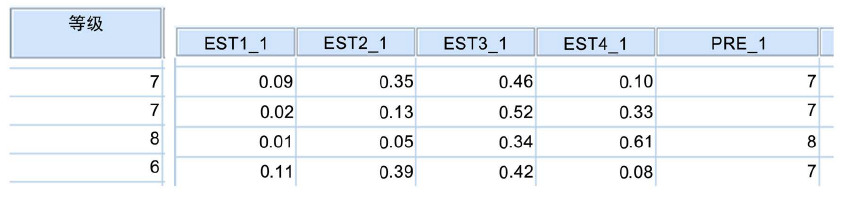# 基于多项有序逻辑回归的大学生数学成绩影响因素分析Analysis of Influencing Factors on College Students’ Mathematics Scores Based on Multiple Ordered Logistic Regression

DOI: 10.12677/ASS.2019.87170, PDF, HTML, XML, 下载: 377  浏览: 848

Abstract: By using the method of multiple ordered logistic regression analysis for modeling, through the usual learning situation, college students’ performance rating has been predicted and analyzed. The results show that plan for postgraduate entrance examination, careful listening in class and mathematics result of college entrance examination will have a greater impact on college students’ math scores tending to be excellent. Consciously doing homework and taking notes have no significant effect on the final math scores of college students, while properly previewing before class helps in the scores. At the same time, the model prediction accuracy can reach 51.69%.

1. 引言

2. 逻辑回归分析的假设检验

a. 因变量：等级。

a. 在达到最大步骤对分次数后，无法进一步增加对数似然值。

b. 卡方统计量的计算基于广义模型最后一次迭代得到的对数似然值。检验的有效性是不确定的。

c. 联接函数：Logit。

3. 多项有序逻辑回归分析Table 3. Model fitting information

$\begin{array}{l}\mathrm{ln}\left(\frac{{\gamma }_{1}}{1-{\gamma }_{1}}\right)=5.126-0.079高考成绩-\left(0.54文理科-2.589考研+\cdots -0.34作业批改情况\right)\\ \mathrm{ln}\left(\frac{{\gamma }_{2}}{1-{\gamma }_{2}}\right)=8.536-0.079高考成绩-\left(0.54文理科-2.589考研+\cdots -0.34作业批改情况\right)\\ \mathrm{ln}\left(\frac{{\gamma }_{3}}{1-{\gamma }_{3}}\right)=12.254-0.079高考成绩-\left(0.54文理科-2.589考研+\cdots -0.34作业批改情况\right)\end{array}$Figure 1. Partial diagram of the prediction results of college students’ mathematics grades

EST1_1、EST2_1、EST3_1、EST4_1代表四个成绩等级的预测概率。其中第一行中EST3_1概率最大，为0.46，对应的PRE_1为7 (良好)，因变量成绩等级的观察值也为7 (良好)，此时模型准确地预测了因变量。而第四行中EST3_1最大，预测的PRE_1为7 (良好)，而因变量的实际的观察值为6 (及格)，此时模型没有准确地预测因变量。Table 4. Rank*PRE_1 Predicted response category cross tabulation

4. 结论

NOTES

*通讯作者。

  肖婕, 宁静, 缪柏其, 等. 影响学生成绩各因素的评价[J]. 中国高等教育评估, 2001(1): 40-43.  王亮红, 宋代清, 徐娜. 聚类分析在学生成绩分析中的应用[J]. 东北电力大学学报, 2009, 29(4): 35-38.  白春玲, 樊顺厚, 刘军利, 等. 学生学习成绩相关性的研究[J]. 数学的实践与认识, 2009, 39(23): 48-55.  宁静, 肖婕, 缪柏其, 等. 高考成绩与大学成绩的相关性研究[J]. 高等理科教育, 2001(3): 46-50.